• 前言：之前的文章（高维数据中特征筛选方法的思考总结——单变量分析筛选法）中，对单变量分析筛选变量进行了初步考量，本文将进一步总结多变量分析筛选法。由于本文处摘录网上的博客，只是进行了归纳整理，因此...
前言：之前的文章（高维数据中特征筛选方法的思考总结——单变量分析筛选法）中，对单变量分析筛选变量进行了初步考量，本文将进一步总结多变量分析筛选法。由于本文多处摘录网上的博客，只是进行了归纳整理，因此笔者将本文定性为转载类（转载请注明出处：https://blog.csdn.net/fjsd155/article/details/93754257）。

多变量分析方法根据建模特点可以分为线性降维和非线性降维。线性降维主要是 LASSO 和 PLS。非线性降维包括：XGBoost（GBDT的高效实现）、Random Forest 等。其实个人感觉CNN应该也是可以进行非线性降维的（但是目前CNN处理“非序列数据”并没有优势，笔者有个预处理的设想，准备尝试一下拓宽CNN的适用范围）。另外SVM这种强大的机器学习方法，似乎只能用来建模而不能筛选特征。

LASSO和PLS都是线性模型的降维方法，也就是说，这两种方法甚至包括上述单变量降维的方法，最终筛选的变量都是为了建立线性模型而准备的，而不能孵育出非线性模型（如存在交互作用的模型、复杂的SVM模型、决策树类模型以及复杂的神经网络模型）。非线性模型的生物学解释性很差（黑箱模型），一般不推荐使用。但是若强行想构建非线性模型，则仅采用上述线性降维的方法是不够理想的，需要采用非线性降维。

注意一个问题的存在：我们筛选特征往往基于训练数据（选择超参数或者最终建模常常会根据交叉验证，但是变量的筛选却常常只是基于训练集）。在训练数据中，变量的贡献程度越大，并不意味着这个变量越有价值。可能某些变量在模型中表现很普通，但是始终十分稳定（经得起考验）；而有些变量在训练数据中表现很好，但外推性却较弱，表现波动大。因此变量的选择有必要综合训练数据和验证数据（如综合CV或Bootstrapping筛选变量）。最后再由独立测试数据进行检验。

筛选特征及建模的科学观念：模型的可重复性（多批数据）＞大样本建模＞模型的准确性。有人提出（貌似是范剑青老师等人提出），“针对一个统计方法，统计准确性、模型可解释性和计算复杂性是衡量其好坏的三个重要指标。”

（下面部分内容参考自：

一些变量筛选方法——1、综述

一些变量筛选方法——2、《An Introduction to Statistical Learning with R》上的数据降维方法

一些变量筛选方法——3、部分其它变量筛选方法）

刚刚从线性与否的角度对降维方法进行了分类概述。另外，An Introduction to Statistical Learning with R 这本书中将筛选变量的方法分为：子集选择法（Subset Selection）、系数压缩法（Shrinkage）、映射降维法（Dimension Reduction）。

子集选择法

子集选择法分为最优子集选择、逐步筛选法等，这部分方法依赖于下述模型评判指标：

Mallows 提出运用Cp去评估一个以普通最小二乘法(Ordinary Least Square或OLS)为假设的线性回归模型的优良性，从而用于模型选择。
日本学者 Akaike 在1974年基于极大似然方法提出了AIC准则，它建立在熵的概念基础上，可以权衡所估计模型的复杂度和此模型拟合数据的优良性。
Schwarz 在Bayes方法的基础上提出BIC准则。与AIC相比，BIC加强了惩罚力度，考虑了样本量，从而在选择变量进入模型上更加谨慎。
Seymour Geisser 提出了交叉验证法（Cross validation)，利用交叉验证结合最小平方误差的方法，是一种在没有任何前提假定的情况下直接进行参数估计的变量选择方法。

此外，还可以使用Adjusted R2进行评价来选择特征子集。这些指标的具体含义和使用方法，可参照An Introduction to Statistical Learning with R 或 一些变量筛选方法——2、《An Introduction to Statistical Learning with R》上的数据降维方法。

最优子集法（Best Subset Selection）：其思想是将所有的特征组合都进行建模，然后选择最优的模型（最优的判断依据都是前面叙述的几种指标）。特点是能够找到全局最优但是计算量较大。

逐步筛选法（Stepwise Selection）分为向前逐步回归与向后逐步回归。其主要思想是：每一次迭代都只能沿着上一次迭代的方向继续进行。向前逐步回归是指初始模型只有常数项，然后逐渐添加变量；向后逐步回归是指初始模型包含了所有变量，然后逐渐删除变量。特点是仅关注局部最优（贪心策略）难以保证全局最优（注：向前与向后逐步回归筛选出的变量可能不一样，但其思想完全一样。）

系数压缩法

系数压缩法主要指LASSO。岭回归只能实现系数压缩而不能降维，但是可以通过合理的调参，将系数压缩后对系数进行排序，从而实现降维（但既然LASSO已经还不错了，没必要强行使用岭回归降维）。

LASSO可参考博客：LASSO回归

映射降维法

PLS可参考博客：偏最小二乘法 Partial Least Squares

其他不错的方法

除了上述三大类方法，树结构的方法以及Boosting类的方法也是比较有效的，如：Random Forest、XGBoost（GBDT的高效实现）等。此外，Fan和Li 结合L0与L1范数提出的SCAD (Smoothly Clipped Absolute Deviation)；Fan 提出的SIS（Sure Independence Screening）等。

Random Forest

随机森林模型本身是用于预测的模型，但在预测过程中，可以对变量重要性进行排序，然后通过这种排序来进行变量筛选。

变量重要性评判用Gini指数为标准，针对一棵树中的每个节点 k，我们都可以计算一个Gini指数：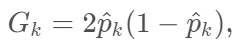其中表示样本在节点 k 属于任意一类的概率估计值。

一个节点的重要性由节点分裂前后Gini指数的变化量来确定：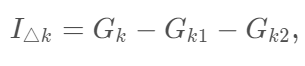和 分别表示 产生的子节点。针对森林中的每棵树，都用上述的标准来递归产生，最终随机抽取样本和变量，产生森林，假设森林共产生 T 棵树。

森林中，如果变量 在第 t 棵树中出现 M 次，则变量 在第 t 棵树的重要性为：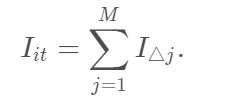则 在整个森林中的变量重要性为：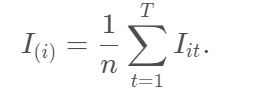最终我们根据变量重要性来选择变量，选择的个数可以用SIS中的方法，选取n−1 或n/logn 个。

XGBoost

GBDT的建模过程是，一步步拟合残差，那么在一步步的拟合的过程中，也便是变量选择的过程（从一定程度上实现了变量的筛选）（这种逐步拟合残差的思想的方法还有PLS）。XGBoost是GBDT的高效实现方法。

GBDT的介绍可参考：GBDT

XGBoost 的介绍可参考：XGBoost

SIS

当遇到超高维数据，即维数P无穷大时，上述的算法会出现问题。针对这类超高维问题，Fan等人提出了SIS的方法。

针对线性回归模型(2)，按照SIS的思想，首先Y为中心化向量，计算Y与每一个自变量 的相关系数，记为

其中,若越大，说明与Y 相关性越强。所以，可以根据  的大小来进行变量选择。对任意的，对  进行从大到小排序，然后取其一个子集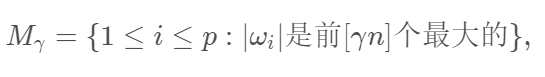其中，n是样本数， 是 的整数部分，进而保证了，与之对应的自变量则入选模型。如果觉得选择  不便于确定，可以选择 n-1 或n/logn。

而关于相关系数，可以选用自己认为合适的。本文后面的模拟选用传统的Pearson相关系数，以及近几年比较火的可用于检验独立的无参数假设的距离相关性（Distance Covariance）（见：一些变量筛选方法——3、部分其它变量筛选方法）。

严格来说，SIS 属于单变量分析方法。

另外，SIS有一些衍生版本，如DC-SIS及Qa-SIS等，其中Qa-SIS好像是可以处理非线性问题的（据说还是“异方差”）。

PDAS

原始对偶激活集算法（Primal Dual Active Set，PDAS）是一个非常新的方法，但做的事情是最优子集选择的事情。其主要思想是引入激活集，对所有的 β 进行批量迭代更新。这个方法的优势在于，可以处理超高维数据（上万维），而最优子集选择一旦超过了50维，基本就完全没办法进行运算。后面我们也将采用PDAS来进行模拟。

PDAS的介绍可参考：一些变量筛选方法——3、部分其它变量筛选方法

另外，有人总结了7种降维方法（七种降维方法）：

缺失值比率 (Missing Values Ratio) ；
低方差滤波 (Low Variance Filter) ；
高相关滤波 (High Correlation Filter)；
随机森林/组合树 (Random Forests)；
主成分分析 (PCA)；
反向特征消除 (Backward Feature Elimination)；
前向特征构造 (Forward Feature Construction)。

本文的总结其实基本上都包含了这些内容。

也有人总结了12种降维方法（在以上7种方法基础上加了5种）（来源：Analytics Vidhya：The Ultimate Guide to 12 Dimensionality Reduction Techniques (with Python codes)，也可参考：12种降维方法终极指南（含Python代码））：

缺失值比率：如果数据集的缺失值太多，我们可以用这种方法减少变量数。
低方差滤波器：这个方法可以从数据集中识别和删除常量变量，方差小的变量对目标变量影响不大，所以可以放心删去。
高相关滤波器：具有高相关性的一对变量会增加数据集中的多重共线性，所以用这种方法删去其中一个是有必要的。
随机森林：这是最常用的降维方法之一，它会明确算出数据集中每个特征的重要性。
向后特征消除：耗时较久，计算成本也都很高，所以只适用于输入变量较少的数据集。
前向特征选择：思路类似于“向后特征消除”。
因子分析：这种方法适合数据集中存在高度相关的变量集的情况。
主成分分析（PCA）：这是处理线性数据最广泛使用的技术之一。
独立分量分析（ICA）：我们可以用ICA将数据转换为独立的分量，使用更少的分量来描述数据。
基于投影的方法：ISOMAP适合非线性数据处理。
t分布式随机邻域嵌入（t-SNE）：也适合非线性数据处理，相较上一种方法，这种方法的可视化更直接。
UMAP：用于高维数据，与t-SNE相比，这种方法速度更快。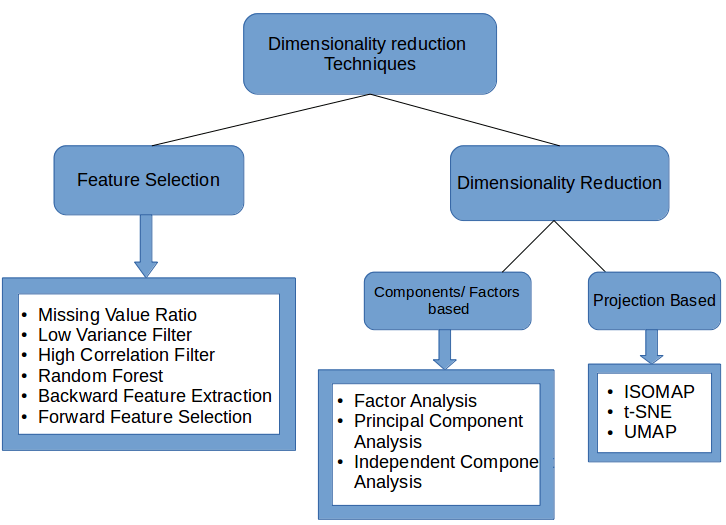之后有空可以再总结下 t-SNE（无监督降维方法，主要用于高维度数据的降维可视化）。

另外，scikit-learn机器学习工具包的官网也有一些特征筛选的方法介绍，有博客基于此进行了介绍（原文：http://dataunion.org/14072.html，但是好像原文访问不了了，可以看看转载的博客，比如：几种常用的特征选择方法，或 干货：结合Scikit-learn介绍几种常用的特征选择方法 ）。

除了这些，还有一些方法也值得一试，如：随机投影(Random Projections)，非负矩阵分解(N0n-negative Matrix Factorization)，自动编码(Auto-encoders)，卡方检测与信息增益(Chi-square and information gain)， 多维标定(Multidimensional Scaling)， 相关性分析(Coorespondence Analysis)，聚类(Clustering)以及贝叶斯模型(Bayesian Models)。

基于聚类的方法，可以参考：

特征筛选（变量聚类proc varclus）

聚类特征变量选取、聚类算法与效果评价简述

特征选择（二）-聚类变换

还有互信息法、模拟退火法以及一些组合策略等，之后再了解一下。参考：

特征选择(2)：特征选择：方差选择法、卡方检验、互信息法、递归特征消除、L1范数

模拟退火筛选变量

特征选择的策略--数据相关性（皮尔逊系数）与gini或者信息熵的的结合

特征选择之基于相关性的特征选择（CFS）

自编码器也是不错的无监督降维方法，是一种神经网络，之后可以研究下。

各种方法的对比评测及代码示例

这部分内容可以参考：

一些变量筛选方法——4、模拟实验

一些变量筛选方法——5、真实数据与总结

一些变量筛选方法——6、代码

参考资料

一些变量筛选方法——1、综述

一些变量筛选方法——2、《An Introduction to Statistical Learning with R》上的数据降维方法

一些变量筛选方法——3、部分其它变量筛选方法

一些变量筛选方法——4、模拟实验

一些变量筛选方法——5、真实数据与总结

一些变量筛选方法——6、代码

LASSO回归

偏最小二乘法 Partial Least Squares

GBDT

XGBoost

七种降维方法

The Ultimate Guide to 12 Dimensionality Reduction Techniques (with Python codes)

Comprehensive Guide on t-SNE algorithm with implementation in R & Python

12种降维方法终极指南（含Python代码）

特征筛选（变量聚类proc varclus）

聚类特征变量选取、聚类算法与效果评价简述

特征选择（一）-维数问题与类内距离

特征选择（二）-聚类变换

展开全文降维 高维数据 生信
• 这段对统计变量操作的代码，曝露在线程环境下，却没有任何同步处理，很容易导致统计数据的不一致问题。  在这个应用中，ReqFailNum++这个操作实际上应该是一个原子操作，它包含了对内存的三个动作“读-修改-写...
      1 问题描述
在一个项目中，需要对发送的请求结果进行统计，开发同事定义了两个全局共享变量CommonUtil.ReqFailNum和ReqNum,分别记录请求失败数和发送的请求数。并在每次发送请求之前都假定该请求会处理失败，先对其累加，直到成功收到200的返回码后，重新修正失败数量。
最后当应用处理请求处于较频繁的阶段时，出现了ReqFailNum最后减为负数的情况，一次正常请求完成时，CommonUtil.ReqFailNum ++;和CommonUtil.ReqFailNum --应该是成对出现的，这个统计值不应该为负数的。
发送请求的代码如下：
private static boolean XMLPost(String content, String sendUrl) throws Exception{
boolean bn = false;

if ( null != content ) {
//初始假设请求发送失败，等待正常返回200后再将失败记录数--
CommonUtil.ReqFailNum ++;

URL url =null;
URLConnection con = null;
OutputStreamWriter out = null;
try {
url = new URL(sendUrl);
con = url.openConnection();
}catch (MalformedURLException e1) {
throw new ConnException("MalformedURLException");
} catch (IOException e) {
throw new ConnException("IOException");
}
con.setConnectTimeout(2000);
con.setDoOutput(true);
con.setRequestProperty("Connection", "keep-alive");
con.setRequestProperty("Pragma:", "no-cache");
con.setRequestProperty("Cache-Control", "no-cache");
con.setRequestProperty("Content-Type", "text/xml");

try {
out = new OutputStreamWriter(con.getOutputStream(), "UTF-8");
out.write(content);
out.flush();
out.close();
} catch (UnsupportedEncodingException e) {
throw new ConnException("UnsupportedEncodingException");
} catch (IOException e) {
String exceptionStr = CommonUtil.stackTraceStr(e);
throw new ConnException("IOException."+exceptionStr);
}finally{
try {
if(out != null){
out.close();
}
} catch (IOException e) {

throw new ConnException("IOException...");
}
}

CommonUtil.ReqFailNum --;
CommonUtil.ReqNum ++;
bn = true;
logger.info("sendUrl:: return 200 ok" );
}
}
return bn;
}
2 错误原因分析
统计变量在并发环境下，开发人员却忽视了其安全问题。由于该方法在Action中调用，客户端的每个请求，都会调用该方法。而Web服务器处理客户端的请求时，对每个请求都创建了一个线程去处理。这段对统计变量操作的代码，曝露在多线程环境下，却没有任何同步处理，很容易导致统计数据的不一致问题。
在这个应用中，ReqFailNum++这个操作实际上应该是一个原子操作，它包含了对内存的三个动作“读-修改-写”，并且结果状态依赖于之前的状态。上述代码，在没有同步的情况下，当两个线程同时执行这行代码时，可能读到的是同一个值，同时+1 ，最终应该是两次累计操作，结果只累加了一次，由于丢失了一次递增操作，最终的统计值就偏差了1。
由于++代码是方法最初的几行，线程同时执行++操作的概率较大，而CommonUtil.ReqFailNum --;是在请求成功处理完成后执行的，这段时间涉及到网络请求，处理时间不确定性较大，所以- -操作同时执行的概率也较低。最终ReqFailNum++丢失的次数会多于ReqFailNum--丢失的次数，从而导致这个共享变量ReqFailNum的值成了负数。
3 解决办法
1）使用锁，将ReqFailNum++或--的操作放在同步代码块中
2）由于是简单的统计变量，可以利用原子变量的特性，使用AtomicInteger或AtomicLong
结论：Web项目中，共享变量的线程安全性容易被忽视，加上数据不一致问题的出现具有偶发、不可预测等因素（本来想截个图的，但是应用目前并发量小，没有出现数据不一致的现象，这也是并发问题隐蔽而不易被发现的原因），为了防患于未然，在项目伊始就应该分析并发因素，让开发人员关注可变状态的线程安全性问题，是非常必要的。

展开全文java 并发 线程安全
• 文章目录一、简单相关性分析1、变量间的关系分析（1）函数关系（2）相关关系i、平行关系ii、依存关系iii、两者关系2、简单相关分析（1）计算两变量之间的线性相关系数i、协方差定义、柯西-施瓦尔兹不等式a、协方差...
文章目录一、简单相关性分析1、变量间的关系分析（1）函数关系（2）相关关系i、平行关系ii、依存关系iii、两者关系2、简单相关分析（1）计算两变量之间的线性相关系数i、协方差定义、柯西-施瓦尔兹不等式a、协方差定义b、柯西-施瓦尔兹不等式ii、Pearson相关系数（样本线性相关系数）注意：数据不服从正态分布时--spearman相关系数iii、ρ=0，相关系数的假设检验a、引入假设检验的原因iv、t-检验的解读a、简历检验假设b、构造 t 统计量，计算相关系数 r 的 t 值c、计算 t 值和 P ，作结论3、深度探讨ρ=0二、多变量相关性分析（一个因变量和多个自变量）1、偏相关或复相关2、意义与用途3、分析方法（1）样本相关系数矩阵、相关系数检验（2）复相关分析（3）决定系数R^2^ (RMSE的介绍)4、小结
一、简单相关性分析
1、变量间的关系分析
变量之间的关系可分为两类：函数关系、相关关系。
（1）函数关系
存在完全确定的关系
（2）相关关系
不存在完全确定的关系：虽然变量间有着十分密切的关系，但是不能由一个或多个变量值精确的求出另一个变量的值，称为相关关系，存在相关关系的变量称为相关变量。
相关变量的关系也可分为两种：平行关系、依存关系
i、平行关系

两个及以上变量间相互影响
相关分析是研究呈平行关系的相关变量之间的关系

ii、依存关系

一个变量变化受另一个变量的影响
回归分析是研究呈依存关系的相关变量之间的关系

iii、两者关系
回归分析和相关分析都是研究变量之间关系的统计学课题，两种分析方法相互结合和渗透2、简单相关分析

相关分析：通过对大量数字资料的观察，消除偶然因素的影响，探求现象之间的相关关系的密切程度和表现形式
主要研究内容：现象之间是否相关、相关的方向、密切程度等，不区分自变量与因变量，也不关心各变量的构成形式
主要分析方法：绘制相关图、计算相关系数、检验相关系数

（1）计算两变量之间的线性相关系数

所有相关分析中最简单的就是两个变量间的线性相关，一变量数值发生变动，另一变量数值会随之发生大致均等的变动，各点的分布在平面图上大概表现为一直线；
线性相关分析，就是用线性相关系数来衡量两变量的相关关系和密切程度
给定二元总体（X,Y）

总体相关系数用ρ表示：cov(X,Y)是x与y的协方差

i、协方差定义、柯西-施瓦尔兹不等式
a、协方差定义
设（X,Y）是二维随机变量，若E[(X-E(X))(Y-E(Y))]存在
则称cov(X,Y)=E[(X-E(X))(Y-E(Y))]，叫X与Y的协方差，也叫X与Y的相关（中心）矩
即X的偏差(X-E(X))与Y的偏差(Y-E(Y))乘积的期望
解读：

当cov(X,Y)>0,X的偏差(X-E(X))与Y的偏差(Y-E(Y))，有同时增加或同时减少的倾向，又由于E(X)和E(Y)都是常数，所以就能够等价于X与Y有同时增加或减少的倾向，称X与Y正相关
当cov(X,Y)<0,X的偏差(X-E(X))与Y的偏差(Y-E(Y))，有X增加Y减少的倾向，或Y增加X减少的倾向，称X与Y负相关
当cov(X,Y)=0,称X与Y不相关，这时可能是X与Y取值毫无关联，也可能是某种特殊的非线性关系

b、柯西-施瓦尔兹不等式
根据柯西-施瓦尔兹不等式变形得ρx,y在区间[-1,1]
ρx,y是没有单位的，因为分子协方差的量纲除以了分母的与分子相同的量纲

两变量线性相关性越密切，|ρx,y|越接近于1
两变量线性相关性越低，|ρx,y|越接近于0
|ρx,y|=0的情况跟上面cov(X,Y)=0情况一样，两变量取值毫无关联或有某种特殊的非线性关系

协方差与相关系数的关系，就像绝对数与相对数的关系（绝对数相当于统计中常用的总量指标；相对数是两个有联系的指标的比值，从数量上反应两个相互联系的现象之间的对比关系。）
ii、Pearson相关系数（样本线性相关系数）
一般用样本线性相关系数来估计总体线性相关系数，数据必须服从正态分布
设（X,Y）是二元总体，简单随机抽样(x1,y1),(x2,y2),……(xn,yn)
样本均值：样本方差：样本协方差：样本相关系数：lxx为x的离差平方和，lyy为y的离差平方和，lxy为x与y离差乘积之和（可正可负）
实际计算可按下面简化：python代码（人的身高体重相关性关系）：
import numpy as np
import matplotlib.pyplot as plt
x=np.array([171,175,159,155,152,158,154,164,168,166,159,164])
y = np.array([57,64,41,38,35,44,41,51,57,49,47,46])
np.corrcoef(x,y)
plt.scatter(x,y)
plt.show()

结果：
array([[1.        ,0.95930314],
[0.95930314,1.        ]])

r>0，则体重和身高呈正相关性
注意：数据不服从正态分布时–spearman相关系数
皮尔森相关系数只能用于分析服从正态分布的连续变量的相关性，对于不服从正态分布的变量，可采用Sperman秩相关系数进行相关性分析。
Sperman秩相关系数，也称等级相关系数。如果数据中没有重复值， 并且当两个变量完全单调相关时，斯皮尔曼相关系数则为+1或−1。
计算逻辑：对两个变量的取值按照从小到大顺序编秩，如果两个值大小相等，则秩次为(index1+index2)/2,
不管Pearson还是spearman，都使用pandas中的corr()函数
iii、ρ=0，相关系数的假设检验
a、引入假设检验的原因

r与其他统计指标一样，也会有抽样误差。从同一总体内抽取若干大小相同的样本，各样本的样本相关系数总会有波动。即根据样本数据是否有足够的证据得出总体相关系数不为0的结论（判断得出的结论是否准确的，不是假的）
要判断不等于0的r值是来自总体相关系数ρ=0的总体，还是来自ρ不等于0的总体，必须进行显著性检验
因为样本间没有线性相关性，可能会杂乱无章，也可能呈现出一些非线性关系（更高阶的关系pearson相关系数不能表示出来）
所以r的显著性检验可以用双侧 t 检验来进行

iv、t-检验的解读
a、简历检验假设b、构造 t 统计量，计算相关系数 r 的 t 值此 t 近似服从t(n-2)分布，如果数据严格服从二元正态分布Γ是gamma函数，F1(a,b;c;d)是高斯超几何函数
当总体相关系数ρ=0时（假定两个随机变量时正态相关的），
样本相关系数r的密度函数为：B是beta函数，此密度函数碰巧就是统计量 t ，就是自由度为n-2的 t 分布；
c、计算 t 值和 P ，作结论
R语言中有cor.test()函数
相关系数的显著性是与自由度（n-2）有关，也就是与样本数量n有关。
样本量小，相关系数绝对值容易接近于1，样本量大，相关系数绝对值容易偏小；
所以，我们要拿到充分大的样本，就能把相关系数r作为总体相关系数ρ，这样就不必关心显著性检验的结果了。
3、深度探讨ρ=0
Pearson相关系数无法度量非线性关系的强度。
二、多变量相关性分析（一个因变量和多个自变量）
多变量基于双变量
1、偏相关或复相关

简单相关：研究两变量之间的关系
偏相关或复相关：研究三个或者三个以上变量的关系

2、意义与用途
有些时候，我们只想了解两个变量之间是否有线性相关关系，并不想拟合建立它们的回归模型，也不需要区分自变量和因变量，这时可用相关性分析。
3、分析方法
（1）样本相关系数矩阵、相关系数检验
设x1,x2…xn，来自正态总体Np(u，σ2)容量为n的样本，其中每个样本x有p各观测
分别计算两两样本之间的简单相关系数rij，它们构成的矩阵就是：由于每个变量跟自己的相关系数就是1，即：其中，（rij)pxp就是两个变量的简单相关系数R语言中，使用cor(x) 得到相关系数矩阵，corr.test(x)进行相关系数检验（得到t检验矩阵），Probability values得到p值（置信度）矩阵
（2）复相关分析

实际分析中，一个变量(y)往往要受到多种变量（x1,x2,…x4）的综合影响，
所谓复相关，就是研究多个变量同时与某个变量的相关关系
度量复相关程度的指标是复相关系数
多个变量同时与某个变量的相关关系不能直接测算，只能通过间接测算

复相关系数的计算：
设因变量y，自变量x1,x2,…xp,构造一个线性模型为：
y=b0+b1x1+…+bpxp+ε
y帽=b0+b1x1+…+bpxp
对y与x1、x2…xp作相关分析，就是对y与y帽作简单相关分析
记：

ry.x1…xp为y与x1,x2…xp的复相关系数
ry.y帽为y与y帽的简单相关系数

ry.x1…xp的计算公式：复相关系数常用于多元线性回归分析中，我们希望知道因变量与一组自变量之间的相关程度，即复相关，复相关系数反映了一个变量与另一组变量的密切程度。
假设检验:
与多元回归的方差分析一样
综上：（3）决定系数R2 (RMSE的介绍)
在复相关系数中，根号里面的比值其实说明了回归平方和与总离平方和的比值，反应了回归贡献的百分比
把复相关系数两边平方一下就能得到决定系数决定系数用于评价多元回归方程、变量选择、曲线回归方程拟合的好坏程度中。
注意：

R2 是相关性的度量，并不是准确性的度量
R2 依赖于y的波动程度（样本方差），这会使得我们看待模型的好坏有着巨大影响，例如，假设测试集y的方差是4.2，如果一个模型的RMSE=1，R2 大致为76%，但是另一个测试集的方差是3，R2 则变为67%。这样模型的好坏就决定于测试集的波动程度，所以这个十分不靠谱
不明白上面的话，可以再看一个例子，如果我们建立了一个模型预测广州房价，如果测试集中广州房屋售价的波动范围较大——方差较大（40万-几千万），因为方差大，所以很可能导致 R2 也比较大（假设 80%），但 RMSE可能十万，这对于广州房价预测来说是一个很糟糕的预测范围。

什么是RMSE：
RMSE 是回归问题的性能指标，衡量的是预测值与真实值之间的差距，是测量预测误差的标准差举例子： RMSE 等于 50000，根据【3σ 准则】意味着：
大约 68% 的预测值位于真实值的 50000元（1σ）以内，
大约 95% 的预测值位于真实值的 100000元 （ 2σ）以内，
大约 99.7% 的预测值位于真实值的 150000元内 （ 3σ ）以内
4、小结
可以看出多变量相关分析跟回归分析的关系很密切，多变量相关分析能为回归分析服务，因为要具有相关性才有做线性回归拟合的价值


展开全文• 变量的关系6.1 联合概率分布6.2变量的独立性6.3 变量的相关性6.4 上证指数与深证成指相关性分析 统计分析是可以帮助人们认清、刻画不确定性的方法。总体是某一特定事物可能发生结果的集合，随机变量(Random ...
目录1. 概率与概率分布1.1离散型随机变量1.1.1概率质量函数(PMF)1.1.2 累计分布密度函数(CDF)1.1.3 Python的实现1.2 连续型随机变量2. 期望值与方差3. 二项分布3.1二项分布概述及其与伯努利分布的差别3.2 Numpy生成二项分布随机数3.3 二项分布的PMF与CDF3.3.1 PMF及其图像绘制3.3.2 CDF及其图像绘制3.3.3 二项分布在金融市场的应用4. 正态分布4.1 正态分布概述4.2 Python正态分布相关函数4.3 正态分布在金融市场的应用5. 其他连续分布5.1 卡方分布5.2 t分布5.3 F分布6. 变量的关系6.1 联合概率分布6.2变量的独立性6.3 变量的相关性6.4 上证指数与深证成指相关性分析
统计分析是可以帮助人们认清、刻画不确定性的方法。总体是某一特定事物可能发生结果的集合，随机变量(Random Variable) 则是一个不确定事件结果是数值函数(Function)。也就是说，把不确定事件的结果用数值来表述，即得到随机变量。随机变量又分为离散型随机变量(Discrete Random Variable) 和 连续型随机变量(Continuous Radom Variable)。1. 概率与概率分布
概率(Probability)是用于刻画事物不确定性的一种测度(Measure)，根据概率的大小，我们可以判断不确定性的高低。
1.1离散型随机变量
1.1.1概率质量函数(PMF)
假设X是一个离散型随机变量，其所有可能取值为集合{ak}，k=1,2,…,我们定义X的概率质量函数(Probability Mass Function) 为：
$\displaystyle {f_X}\relax{(a_k)}=P\{X=a_k\} , k=1,2,\cdots$
概率质量函数可以量化地表达随机变量X取每个数值可能性的大小
1.1.2 累计分布密度函数(CDF)
对于离散型随机变量，累计分布函数可以用概率质量函数累加来获得：
$\displaystyle {F_X}\relax{(a)}=P\{X\leq a\}=\sum_{i:a_i\leq a}{f_X}\relax{(a_i)}$
1.1.3 Python的实现
在Python中我们可以通过Numpy包的random模块中的choice()函数来生成服从待定的概率质量函数的随机数。
choice()函数：

choice(a, size=None, replace=True, p=None)
参数a: 随机变量可能的取值序列。
参数size: 我们要生成随机数数组的大小。
参数replace: 决定了生成随机数时是否是有放回的。
参数p：为一个与x等长的向量，指定了每种结果出现的可能性。

计算频数分布使用value_counts()函数
# 以数组形式
import numpy as np
import pandas as pd

RandomNumber=np.random.choice([1,2,3,4,5],\
size=100, replace=True,\
p=[0.1,0.1,0.3,0.3,0.2])
pd.Series(RandomNumber).value_counts() # 计算频数分布value_counts()函数
pd.Series(RandomNumber).value_counts()/100  #计算概率分布

结果呈现：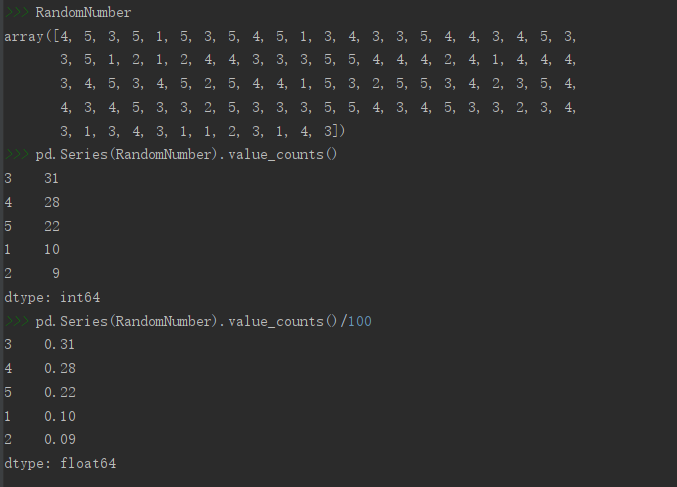增大size参数，即生成随机数的数目，得到的结果则会更接近设定的概率。

1.2 连续型随机变量
若随机变量X是连续的，我们则不再能通过概率质量函数来刻画随机变量只随机性，对任意ak都有$\displaystyle P\{X=a_k\}=0$。
连续型随机变量没有PMF。对于连续型随机变量，累积分布函数
$\displaystyle {F_X}\relax{(a)}=P\{X\leq a\}$可以表达为：
$\displaystyle {F_X}\relax{(a)}=\int _{-\infty}^{a}{f_X}\relax{(x)}dx$
其中$\displaystyle {f_X}=\frac{dF_X(x)}{dx}$，被称作概率密度函数
(Probability Density Function)(PDF)，X的取值落在某个区间的概
率可以用概率密度函数在这个区间上的积分来求得。
算法逻辑：

使用stats模块kde包中的gaussian_kde()可以估计数据的概率密度，在其中传入我们要统计的Series类型的变量名即可，得到的是一个“scipy.stats.kde.gaussian_kde”类型的对象，我们暂先给其命名为density。
然后设定好分割区间，设为数组格式，暂将该对象命名为bins。
注意，这次与以往绘图不同，这次是以研究的对象数据为行进行绘图。
使用上边得到的数据类型density，以 density(bins)格式的语法可以得到一个以设定的分割区间为界限的概率密度数组，让将density(bins)这个对象作为行传入plot即可。
如果要绘制累计分布函数图，则只需在上边的density(bins)对象后再调用一下cumsum()函数，对数组数据进行累加后在传入即可。

以2020年沪深300指数(399300.SZ)的收益率(日涨跌幅)数据为例：
# 调取数据
import numpy as np
import tushare as ts
import pandas as pd
token = 'Your Token'   # 输入你的接口密匙，获取方式及相关权限见Tushare官网。
pro = ts.pro_api(token)
df = pro.index_daily(ts_code='399300.SZ')
df = df.sort_index()

# 实现概率分布
import matplotlib.pyplot as plt
from scipy import stats
density = stats.kde.gaussian_kde(df.pct_chg['2020']) #研究数据格式化
bins=np.arange(-5,5,0.02)  # 设定分割区间

plt.rcParams['font.sans-serif'] = ['SimHei']
plt.rcParams['axes.unicode_minus'] = False
plt.subplot(211)
plt.plot(bins,density(bins))
plt.title('沪深300指数2020年收益率序列概率密度曲线图')
plt.subplot(212)
plt.plot(bins,density(bins).cumsum())
plt.title('沪深300指数2020年收益率序列累积分布函数图')
plt.show()

结果展示如下：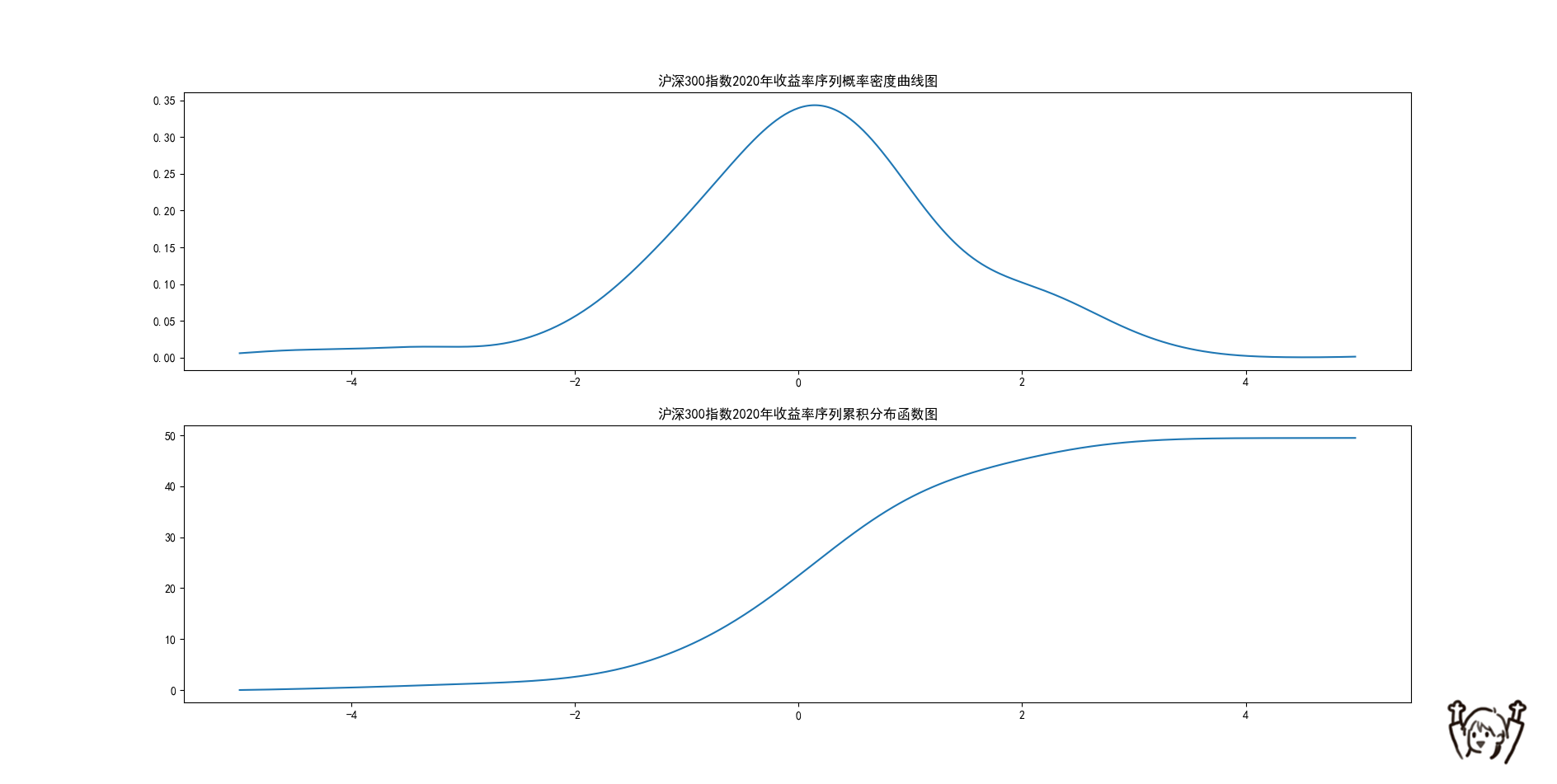2. 期望值与方差
可以用样本数据的平均值来刻画样本的中心位置，期望(Expectation)是随机变量所有可能取的结果的均值，用来呈现总体的中心位置。对于离散型随机变量，期望是该随机变量所有可能结果的取值与其概率的乘积之和：
$\displaystyle E\relax{(X)}=\sum_ka_kf_X\relax{(a_k)}=\sum_ka_kP\{X=a_k\}$
方差(Variance)则是:
$\displaystyle Var\relax{(X)}=E\left[X-E\right]^2=\sum_k\left[a_k-E(X)^2\right]P\{X=a_k\}$
对于一类特殊的离散型随机变量：伯努利(Bernoulli Random Variable)随机变量，伯努利随机变量X只能取到两个值，0或者1，对应的概率质量函数为：
$\displaystyle f_X\relax{(a)}=P\relax{(X=a)}=\begin{cases}p,&a=1 \\1-p,&a=0\end{cases}$
伯努利随机变量的期望值$\displaystyle E(X)=p$
方差为$\displaystyle Var(X)=(1-p)·p$
若随机变量X为连续型随机变量，其概率密度函数为$\displaystyle f_X$，则期望为：$\displaystyle E(X)=\int_{-\infty}^{\infty}af_X\relax{(a)}d\relax{a}$
方差为：$\displaystyle Var\relax{(X)}=\int_{-\infty}^{\infty}\left[a-E\relax{(X)}\right]^2f_X\relax{(a)}d\relax{a}$
随机变量可能的取值有很多(比如连续型随机变量的取值为无穷)，但其观测值(实际值)个数有限，因此现实中随机变量的概率分布、期望、方差等特征值通常是不可知的，推断统计就是透过其观测值的集合——样本数据来刻画这些特征的。

3. 二项分布
3.1二项分布概述及其与伯努利分布的差别
研究伯努利分布时，我们关注的期望是进行一次试验结果的期望值，这样的结果有两种情况，所以伯努利分布也称“两点分布”。
而研究二项分布时，我们关注的是n次试验的结果，这样的结果有多种组合。
为直观说明，假设二点分布结果0的概率为0.4，结果为1的概率为0.6；
二项分布结果为0的概率为0.4，结果为1的概率为0.6，且进行十次。
则：
n = 10
p1 = 0.6
p2 = 0.6
plt.rcParams['font.sans-serif'] = ['SimHei']
plt.rcParams['axes.unicode_minus'] = False
plt.subplot(221)
plt.bar(['0','1'], [1-p1,p1], width=0.5)
plt.title("二点分布PMF")
plt.subplot(222)
plt.plot(['0','0','1','1',' '], [0, 0.4, 0.4, 1.0,1.0])
plt.title("二点分布CDF")
plt.subplot(223)
b = [stats.binom.pmf(i,10,0.6) for i in range(0,11)]
plt.bar([str(i) for i in range(0,n+1)],b)
plt.title('二项分布PMF')
plt.subplot(224)
plt.title("二项分布CDF")
c = [str(i//2) for i in range(0,2*(n+1))]
c.append('')
d = [stats.binom.cdf(i//2,10,0.6) for i in range(0,22)]
d.insert(0,0)
plt.plot(c,d)
plt.show()

展示结果：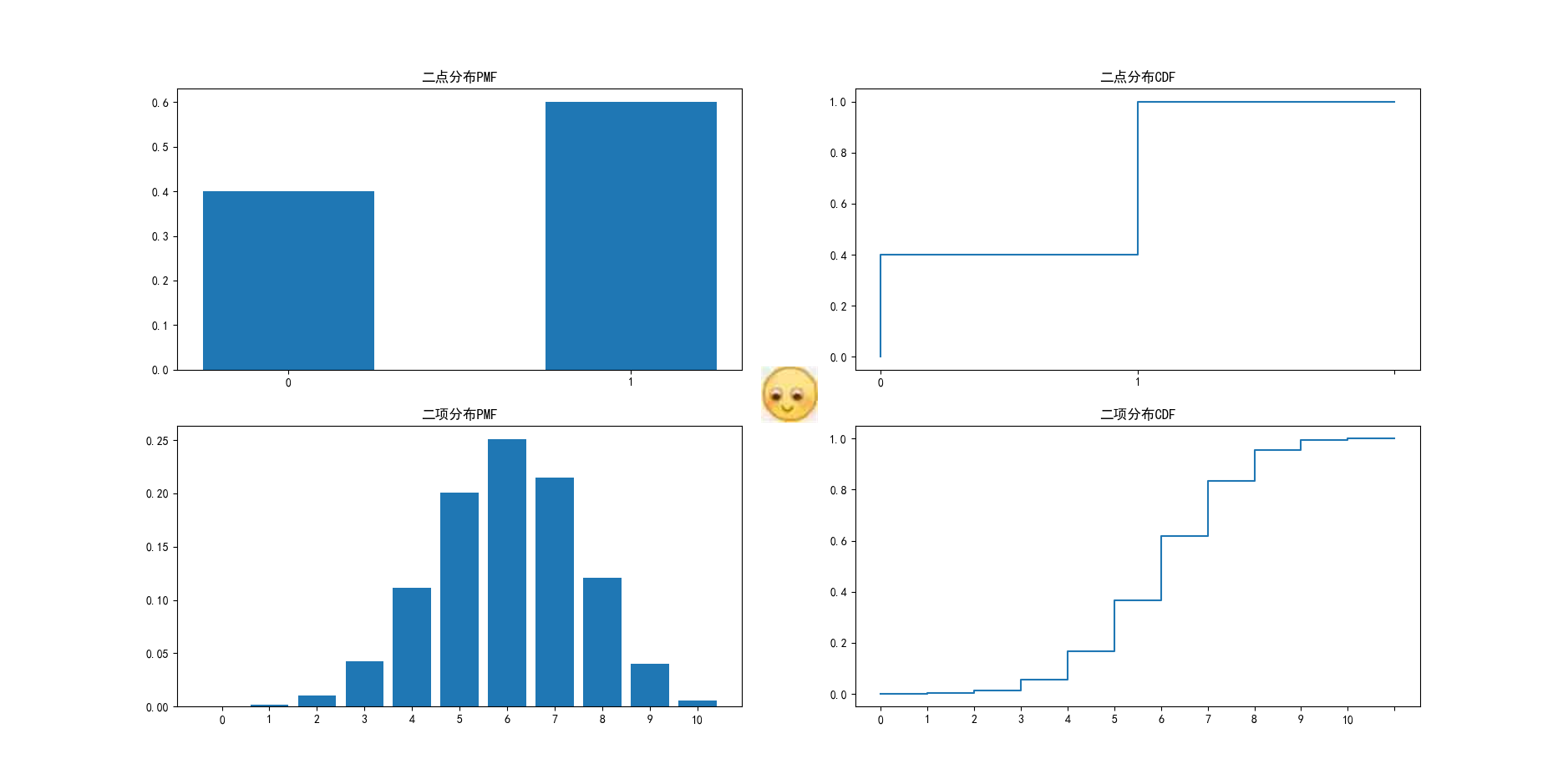当np≥10，n(1-p)≥10都满足时，二项分布可以近似为正态分布。
3.2 Numpy生成二项分布随机数
在Numpy库中可以使用binomial()函数来生成二项分布随机数。
形式为：binomial(n, p, size=None)
参数n是进行伯努利试验的次数，参数p是伯努利变量取值为1的概率，size是生成随机数的数量。
np.random.binomial(100, 0.5, 20)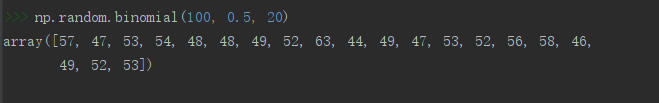3.3 二项分布的PMF与CDF
3.3.1 PMF及其图像绘制
# 求100次试验，20次成功的概率，p=0.5
stats.binom.pmf(20,100,0.5)
# 求100次试验，50次成功的概率,p=0.5
stats.binom.pmf(50,100,0.5)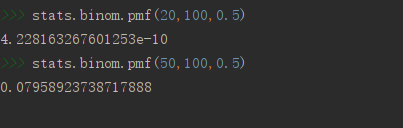图像绘制
#传入时n+1是因为10次实验有十一种可能的结果组合。
n=10   # 十次试验
p=0.5
plt.rcParams['font.sans-serif'] = ['SimHei']
b = [stats.binom.pmf(i, n, p) for i in range(0,n+1)]
plt.bar([str(i) for i in range(0,len(b))],b)
plt.title('X~B({},{})二项分布PMF'.format(n,p))
plt.show()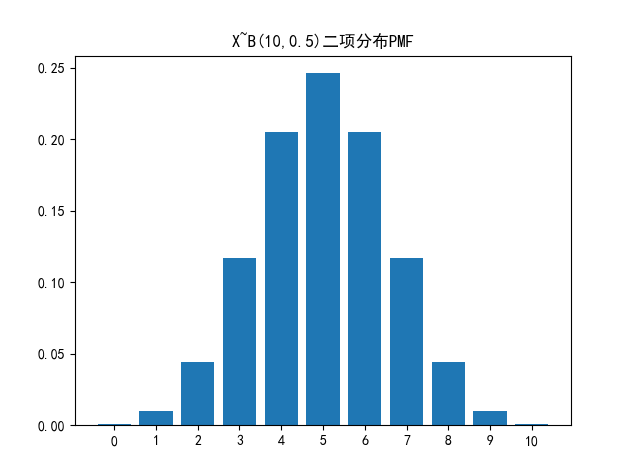3.3.2 CDF及其图像绘制
可以直接用pmf()函数解决这个问题。
#stats.binom.pmf函数很神奇，传入的第一个参数是数字(指定伯努利试验成功的次数)，生成结果就也是一个数字。如果传入的第一个参数是数组，则会将会以该数组的shape输出其中每个数字成功次数的条件下，对应的概率。
dd = stats.binom.pmf(np.arange(0, 21, 1), 100, 0.5)
dd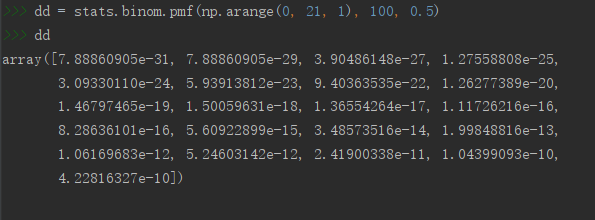# 然后对数组求和即求出小于等于20次发生这一事件的概率
dd.sum()此外，也可以用binom中的cdf()函数来直接解决这个问题
# 依然100次试验成功20次，每次p=0.5
stats.binom.cdf(20,100,0.5)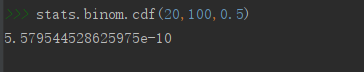图像绘制
n=10   # 十次试验
p=0.5
plt.rcParams['font.sans-serif'] = ['SimHei']
plt.title('X~B({},{})二项分布CDF'.format(n,p))
c = [str(i//2) for i in range(0, 2*(n+1))]
c.append('')
d = [stats.binom.cdf(i//2, n, p) for i in range(0, 22)]
d.insert(0,0)
plt.plot(c,d)
plt.show()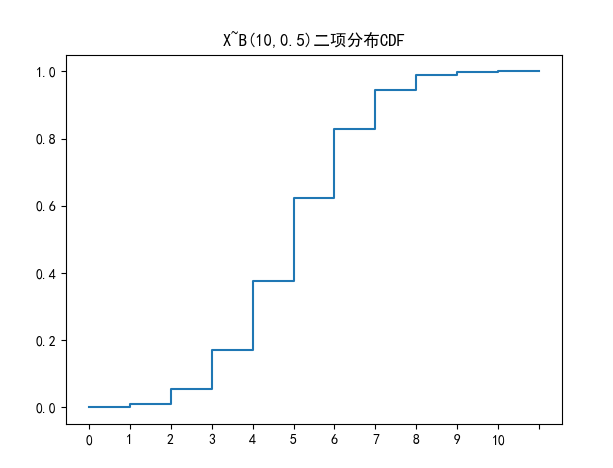3.3.3 二项分布在金融市场的应用
二项分布常常用于描述金融市场中只有两个结果的重复事件。
如研究平安银行股票2020年数据：
# 导入相关模块
import numpy as np
import tushare as ts
import pandas as pd
from scipy import stats
# 设定好接口  注意这句不能照抄，需要输入自己的接口密匙
token = 'Your token'   # 输入你的接口密匙，获取方式及相关权限见Tushare官网。
pro = ts.pro_api(token)
# 获取数据
df = pro.daily(ts_code='000001.SZ')  # daily为tushare的股票日线数据接口。
df = df.sort_index()
ret = df.pct_chg['2020']

# 估算平安银行股价上涨的概率
p = len(ret[ret > 0]) / len(ret)
print(p)

# 估计十个交易日中，平安银行有六个交易日上涨的概率
prob = stats.binom.pmf(6,10,p)
print(prob)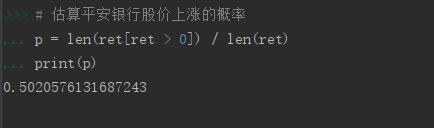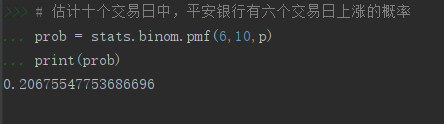4. 正态分布
4.1 正态分布概述
正态分布(Normal Distribution)又名高斯分布(Gaussiam Distribution)，是人们最常用的描述连续型随机变量的概率分布。在金融学研究中，收益率等变量的分布假定为正态分布或者对数正态分布(取对数后服从正态分布)。因为形状的原因，正态分布曲线也被经常称为钟形曲线。
正态分布分布律： 若随机变量X满足服从一个数学期望为u, 方差为$\displaystyle \sigma^2$的正态分布，记为X ~$\displaystyle(N,\sigma^2 )$，则X的取值范围为($\displaystyle -\infty,+\infty$)，其概率密度为：
$\displaystyle f_X\relax{(x)}=\frac{1}{\sqrt{2\pi\sigma}}exp\left[\frac{-(x-u)^2}{2\sigma^2}\right]$
均值越大图像越靠右，方差越小图像越瘦高
服从正态分布的变量X满足其线性变换aX+b~($\displaystyle au+b,a^2\sigma^2$)。
若令a = $\displaystyle\frac{1}{\sigma}$，b = $\displaystyle\frac{-u}{\sigma}$，即可得到标准正态分布，该过程即标准化，数学式记作：
$\displaystyle Z=\frac{X-u}{\sigma}$ ~ N$\displaystyle(0,1)$
任何正态分布都可以进行标准化，转变成标准正态分布。
4.2 Python正态分布相关函数
正态分布随机数的生成函数是normal()，其语法为：
normal(loc=0.0, scale=1.0, size=None)

参数loc：表示正态分布的均值
参数scale：表示正态分布的标准差，默认为1
参数size：表示生成随机数的数量

# 生成五个标准正态分布随机数
Norm = np.random.normal(size=5)
# 求生成的正态分布随机数的密度值
stats.norm.pdf(Norm)
# 求生成的正态分布随机数的累积密度值
stats.norm.cdf(Norm)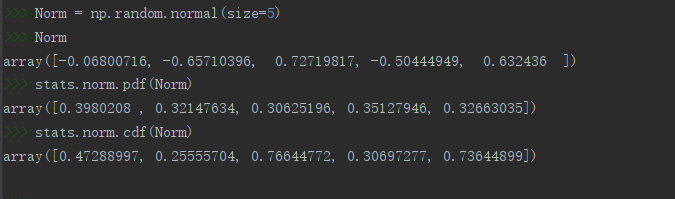绘制正态分布PDF
# 注意这里使用的pdf和cdf函数是norm包里的
u=0
sigma=1
plt.rcParams['font.sans-serif'] = ['SimHei']
plt.title('X~N({},{})正态分布PDF'.format(u,sigma**2))
x = np.linspace(-5,5,100000)  # 设定分割区间
y1 = stats.norm.pdf(x,u,sigma**2)
plt.plot(x,y1)
plt.tight_layout()  # 自动调整子图，使之充满画布整个区域
plt.show()

图像效果：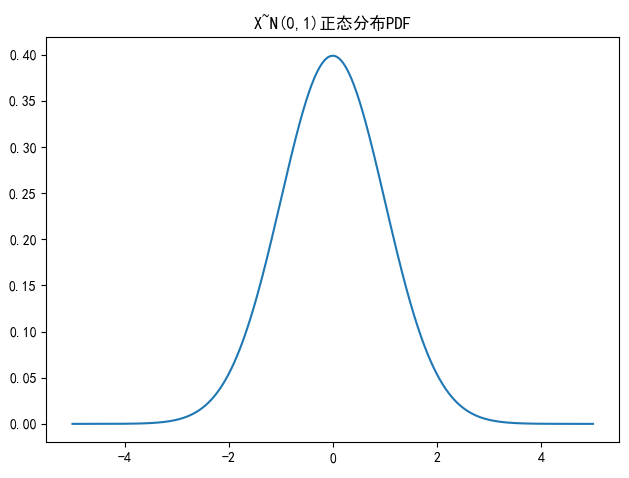绘制正态分布CDF
plt.rcParams['font.sans-serif'] = ['SimHei']
plt.title('X~N({},{})正态分布CDF'.format(u,sigma**2))
x = np.linspace(-5,5,100000)  # 设定分割区间
y2 = stats.norm.cdf(x,u,sigma**2)
plt.plot(x,y2)
plt.tight_layout()
plt.show()

图像效果：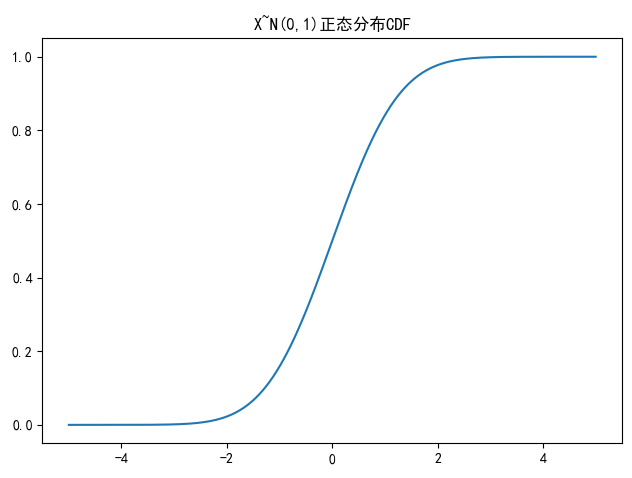4.3 正态分布在金融市场的应用
VAR(Value at Risk)，即在险价值。是指在一定概率水平(α%)下，某一金融资产或金融资产组合在未来特定一段时间内的最大可能的损失，该定义可表达为：$\displaystyle P\{X_t < -VaR\}$ = α%
其中，随机变量Xt为金融资产或金融资产组合在持有期$\displaystyle \Delta t$内的损失，1-α%被叫做VaR的置信水平。
这里用到了ppf() 函数，来获取指定分位数的累积密度值。
假设平安银行股价2020年日收益率序列服从正态分布，下面用Python来求解当概率水平为5%时平安银行股价日收益率在2021年初个交易日的VaR:
# 上边已经定义过ret变量了，为平安银行2020年股价日收益率数据，
# 这里直接接着使用
ret_mean = ret.mean()   # 求均值
ret_variance = ret.var()  # 求方差
# 查询累积密度值为0.05的分位数
stats.norm.ppf(0.05, ret_mean, ret_variance**0.5)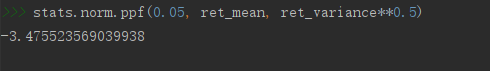也就是说，在2021年的第一个交易日，有95%概率平安银行股票的损失不会超过3.475523569%。
（虽然当天实际跌了3.83，产生了异常值。）

5. 其他连续分布
5.1 卡方分布
若Z1, Z2, … Zn,为n个服从标准正态分布的随机变量，则变量：
$\displaystyle X=Z_1^2+Z_2^2+\cdots+Z_n^2$
服从自由度(Degree of Freedom)为n的卡方分布，
通常表示为$\displaystyle X^2$~$\displaystyle \chi^2$
因为n的取值可以不同，所以卡方分布是一族分布而不是一个单独的分布。根据X的表达式，服从卡方分布的随机变量值不可能取负值，其期望值为n，方差为2n。
plt.plot(np.arange(0, 5, 0.002),\
stats.chi.pdf(np.arange(0, 5, 0.002), 3))
plt.title('卡方分布PDF（自由度为3）')

生成图像如下：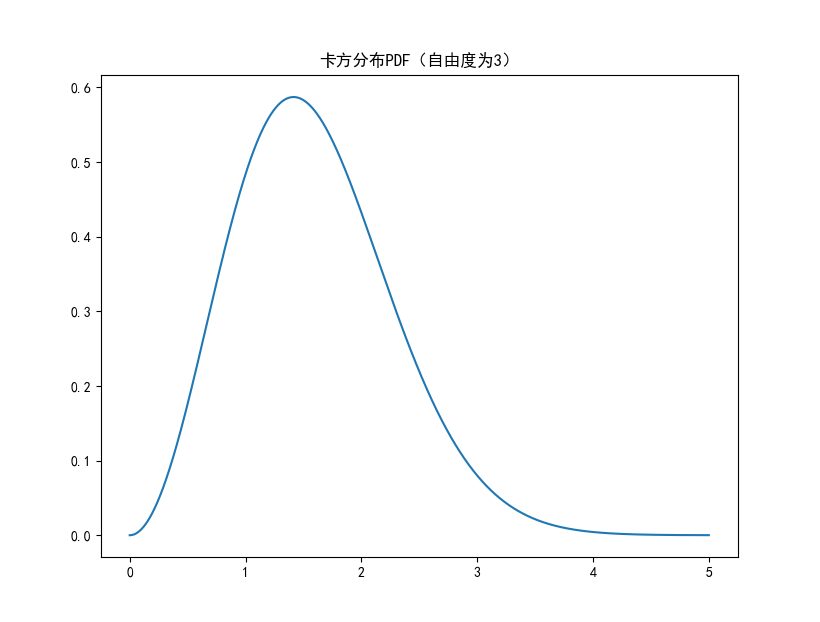卡方分布以0为起点，分布是偏斜的，即非对称的，在自由度为3的卡方分布下，大多数值都小于8，查表可知只有5%的值大于7.82%。

5.2 t分布
若随机变量$\displaystyle Z$ ~ $\displaystyle N(0,1)$，$\displaystyle Y$ ~ $\displaystyle\chi^2(n)$，且二者相互独立，
则变量$\displaystyle X=\frac{Z}{\sqrt{Y/n}}$服从自由度为n的t分布，
可以记作$\displaystyle X$~$\displaystyle t(n)$。
类似卡方分布，t分布也是整整一族，自由度n不同t分布即不同。
t分布变量取值范围为（$\displaystyle -\infty,+\infty$），其期望值与方差存在于否，取值大小均与t分布的自由度有关。

t(1)分布无有限期望值。
t(2)有有限期望值，但方差不存在。
n>2时，t(n)才同时有有限的期望值和方差，其中期望值为0，方差为n/(n-2)，因此自由度越大，变量的方差越小，也就是说分布的离散程度越小。

下边使用Python绘制不同自由度下的t分布的概率分布图：
x = np.arange(-4,4.004,0.004)
plt.plot(x, stats.norm.pdf(x), label='Normal')
plt.plot(x, stats.t.pdf(x,5), label='df=5')
plt.plot(x, stats.t.pdf(x,30), label='df=30')
plt.legend()

结果如图所示：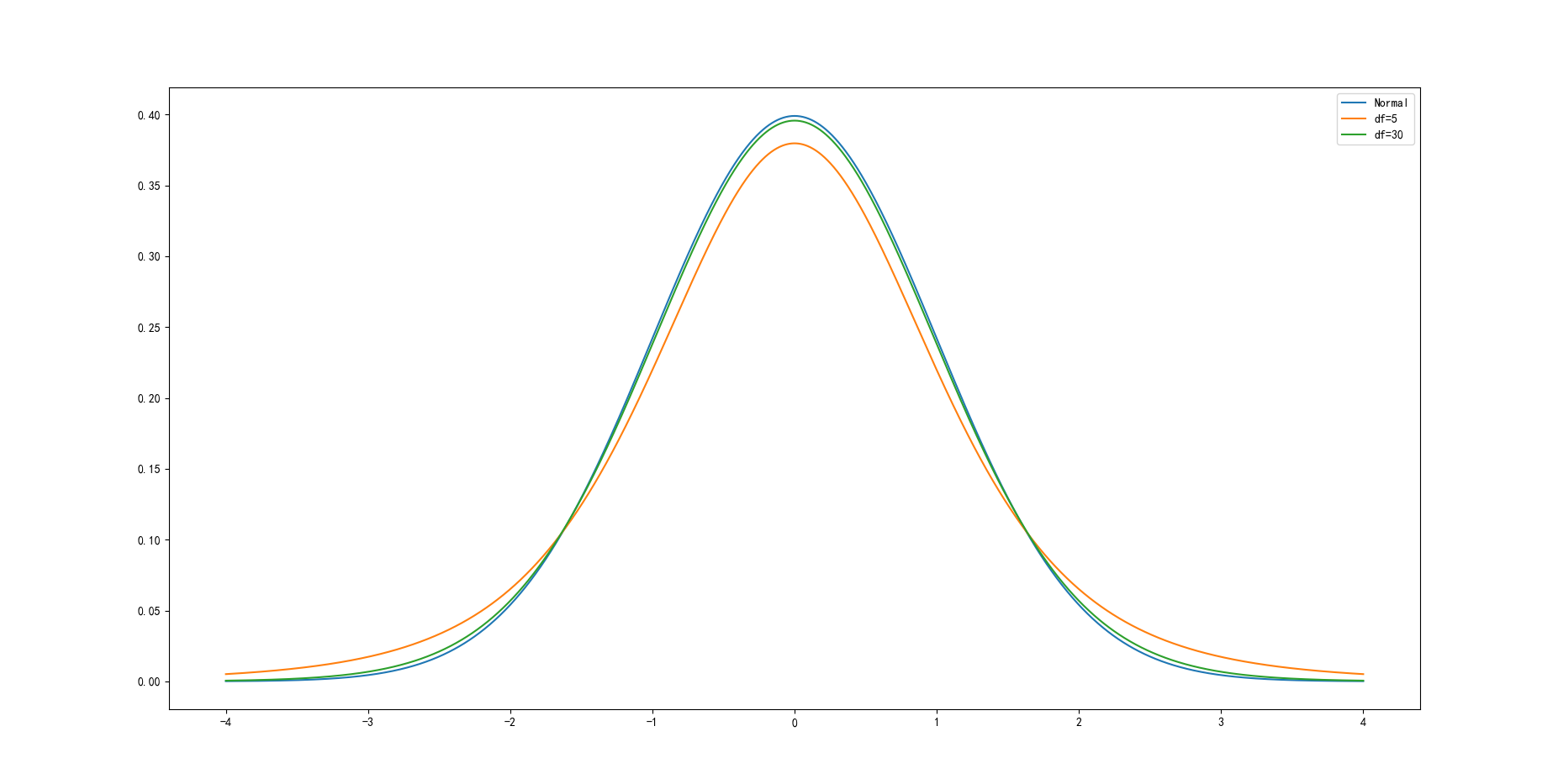t分布的pdf曲线是以0为中心，左右对称的单峰分布，其形态变化与自由度n的大小有关，自由度越小，分布越分散；自由度越大，变量在其均值周围的聚集程度越高，也越接近标准正态分布曲线。
自由度为30时，t分布已经接近标准正态分布曲线。相较于标准正态分布，t分布的密度函数呈现出“尖峰厚尾”的特点。在现实中资产收益率分布往往呈现这种形态，因此t分布在对实际抽样结果的刻画上更为精确。t分布是在推断统计中常用的分布。
————
5.3 F分布
若Z，Y为两个独立的随机变量，且$\displaystyle Z$~$\displaystyle \chi^2(m)$、$\displaystyle Y$~$\displaystyle ~\chi^2(n)$，
则变量$\displaystyle X=\frac{Z/m}{Y/n}$服从第一自由度为m，第二自由度为n的F分布。
记作X~$\displaystyle F(m, n)$
变量X是两个卡方变量(非负)之比，因此X的取值范围也为非负，其期望和方差存在于否取决于第二自由度n。
n > 2时，才存在期望，为$\displaystyle \frac{n}{n-2}$
n > 4时，才存在方差，为$\displaystyle \frac{2n^2(m+n-2)}{m(n-2)^2(n-4)}$
plt.plot(np.arange(0,5,0.002),\
stats.f.pdf(np.arange(0,5,0.002), 4, 40))
plt.title('F分布PDF(df1=4, df2=40)')

它的概率密度函数形态如图所示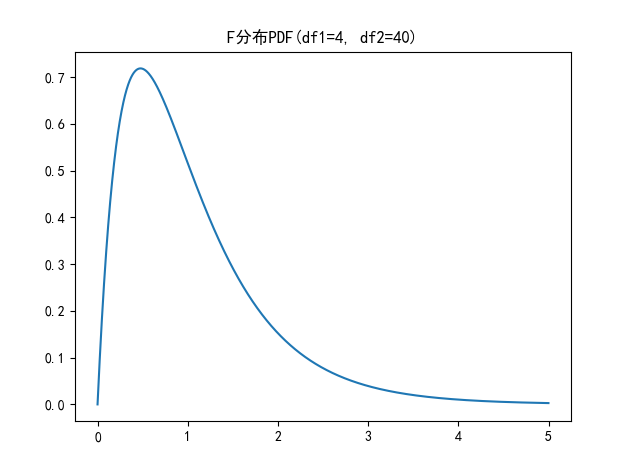6. 变量的关系
我们面对的多个随机变量，之间可能会有互相影响。
6.1 联合概率分布
多个变量之间的联合行为可以用联合概率分布(Joint Probability Distribution)来刻画。
若变量X, Y是离散的，其所有可能取值的集合为{ak}和{bk}, k=1，2，…，
则X，Y的联合概率质量函数(Joint Probability Mass Function)为:
$\displaystyle f_{X,Y}(a,b)=P\{X=a_i 且 Y=b_j\}$,    i,j=1,2…
双变量X和Y的联合累积分布函数(Joint Cumulative Distribution Function)为：   $\displaystyle F_X,Y(a,b)=P\{X \leq a 且 Y \leq b\}$

当X和Y是离散型的：
$\displaystyle F_{(X,Y)}(a,b)=\sum_{i:a_i \leq a} \sum_{j:b_j \leq b} P \{X=a_i且Y=b_j\}=\sum_{i:a_i \leq a} \sum_{j:b_j \leq b}f_{X,Y}(a_i,b_j)$
当X和Y是连续型的：
$\displaystyle F_{(X,Y)}(a,b)=\int_{-\infty}^a\int_{-\infty}^bf_{X,Y}(x,y)dxdy$

其中$\displaystyle f_{X,Y}(x,y)dxdy$是联合概率密度函数(Joint Probability Density Function)>
若已知X和Y的联合概率分布，则X的期望值为：
$\displaystyle E(X)=\sum_i \sum_ja_if_{X,Y}(a_i,b_j)$
$\displaystyle =\sum_i a_i\sum_jf_{X,Y}(a_i,b_j)$
$\displaystyle =\sum_i a_if_X(a_i)$
其中$\displaystyle f_X(a_i)=\sum_jf_{X,Y}(a_i,b_i)$为变量X的边际概率函数(Marginal Probability Function)，代表的是变量X自己的概率分布，所以这里$\displaystyle f_X(a_i)$依然满足$\displaystyle {f_X}\relax{(a_k)}=P\{X=a_k\}$定义式。同理可得$\displaystyle E(Y)=\sum_j b_jf_Y(b_j)$，其形式与单变量的形式无差别。方差求解亦是如此。

6.2变量的独立性
如果随机变量X和Y的联合累积分布函数满足：
$\displaystyle F_{(X,Y)}(a,b)=F_X(a)F_Y(b)$
则称X和Y是独立的，否则二者是相依的。
两变量的独立关系也可以表达成，
$\displaystyle f_{X,Y}(a,b)=f_X(a)f_Y(b)$
对于离散型随机变量，此式等价于
$\displaystyle P\{X=a且Y=b\}=P\{X=a\}P\{Y=b\}$
变量之间的相互独立是众多统计分析的基本假设，也是变量之间的基本关系。

6.3 变量的相关性
随机变量X和Y的协方差(Covariance)可以衡量二者之间的关系，描述的是两随机变量与各自期望的偏差共同的变动状况，表达式为：
$Cov(X,Y)=E\left[(X-E(X))(Y-E(Y))\right]$
协方差为正说明，平均而言变量X、Y与各自期望的偏差呈同方向变动；如果为负，则为反方向变动。
协方差有如下性质：

$Cov(X,Y)=Cov(Y,X)$
$Cov(aX,bY)=abCov(Y,X)$  (a,b是常数)
$Cov(X_1+X_2,Y)=Cov(X_1,Y)+Cov(Y,X_2)$

第二个性质说明，协方差受比例影响，并不能准确地衡量两变量相关性的大小。，不能直接衡量两变量相关性强弱。对协方差进一步处理，清除比例的影响，于是就有了相关系数(Correlation Coefficient):
$\displaystyle \rho_{X,Y}=\frac{Cov(X,Y)}{\sigma_X\sigma_Y}$
相关系数取值范围为[-1,+1]
ρ=0时，说明两个变量是不相关的。
0<ρ<1时， 说明两变量呈正向的线性关系
-1<ρ<0时，说明两变量呈负向的线性关系。
aX和bX的相关性：
$\displaystyle \rho_{aX,bY}=\frac{Cov(aX,bY)}{\sqrt{Var{(aX)}}\sqrt{(Var(bY))}}$
$\displaystyle=\frac{abCov(X,Y)} {|a|\sigma_X|b|\sigma_Y}$
$\displaystyle=\pm \rho_{X,Y}$
如果变量Y是X的线性变换，满足Y=a+bX，则二者之间的相关系数为：‘
$\displaystyle \rho_{X,Y}=\frac{Cov(X,a+bX)}{\sigma_X\sqrt{Var(a+bX)}}$
$\displaystyle \frac{b{Var(X)}}{|b|Var(X)}=\pm1$

6.4 上证指数与深证成指相关性分析
上证指数和深证成指是股票市场常用的反映股票市场情况的指数，我们认为两个指数的日收益率可以存在相关的关系。代码如下：
import numpy as np
import tushare as ts
import pandas as pd
token = 'Your Token'   # 输入你的接口密匙，获取方式及相关权限见Tushare官网。
pro = ts.pro_api(token)
# 深证成指
SZindex = pro.index_daily(ts_code='399001.SZ')
SZindex = SZindex.sort_index()
# 上证指数
SHindex = pro.index_daily(ts_code='000001.SH')
SHindex = SHindex.sort_index()

# 用2020年日收益率数据绘制散点图
plt.rcParams['font.sans-serif'] = ['SimHei']
plt.rcParams['axes.unicode_minus'] = False
plt.scatter(SHindex.pct_chg['2020'],SZindex.pct_chg['2020'])
plt.title('上证指数与深证成指2020年收益率散点图')
plt.xlabel('上证指数收益率')
plt.ylabel('深证成指收益率')
plt.show()

生成图像展示如下：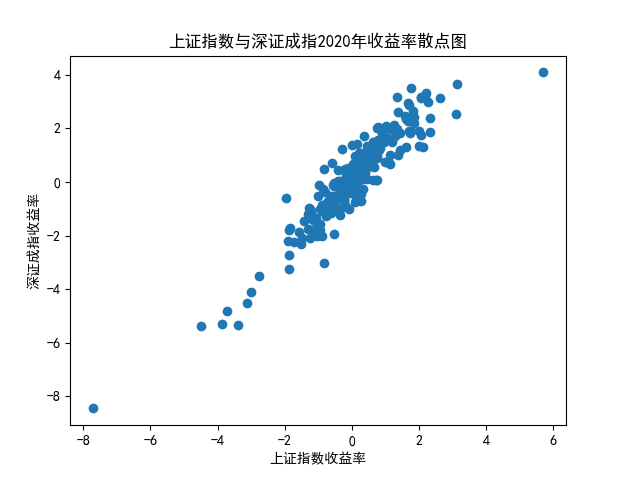# 计算上证综指和深证成指收益率相关系数
SHindex.pct_chg['2020'].corr(SZindex.pct_chg['2020'])相关系数为0.9308847411746248，可以认为二者存在较强的相关性，二者呈现正向的线性关系。展开全文python 统计模型
• 目录标题一元线性回归回归系数的解释核心解释变量和控制变量四类模型回归系数的解释虚拟变量的解释分类的虚拟变量设置含有交互项的自变量容易出现的问题拟合优度 R平方较低怎么办异方差的问题自相关的问题逐步回归...机器学习 python
• 原文链接：http://tecdat.cn/?p=18169 比如说分类变量为是否幸存、是因变量，连续变量为年龄、是自变量，这两者可以做相关分析吗？两者又是否可以做回归分析？ 我们考虑泰坦尼克号数据集，R语言 逻辑回归
• 在这篇文章中，我们将比较LASSO、PLS、Random Forest等多变量模型与单变量模型的预测能力，如著名的差异基因表达工具DESeq2以及传统的Mann-Whitney U检验和Spearman相关。使用骨骼肌RNAseq基因表达数据集，我们将...lasso 机器学习 基因表达式
• Constant, linear, interaction, and squared terms 可点击 上图中的 Export 将所得的参数（beta，rmse）导出 .beta是所得多项式的参数， 例如当model为linear，且只有两个自变量为，多项式公式为：常数 + aX1 + ...keras
• ## 多变量时间序列的预测和建模指南

万次阅读 多人点赞 2019-06-24 12:35:01
1.单变量多变量时间序列 本文假定读者熟悉单变量时间序列的性质，以及用于预测的各种技术。由于本文将重点讨论多变量时间序列，因此我建议您阅读以下文章，这些文章可以作为对单变量时间序列的良好介绍： 时间...
• 个数据集上的预测错误的均值（期望）。 对于给定的假设集，我们可以计算出模型的 真实错误（true error） ，也称泛化错误、测试错误 ∑ x E P [ ( y − h ( x ) ) 2 ∣ x ] P ( x ) , \sum_{\textbf{x}}E_P[(y...机器学习
• 最近我被问到我的 - [R和Stata的软件包是否能够适应协变量之间的非线性关系。答案是肯定的，在这篇文章中，我将说明如何做到这一点。 为了说明，我们将模拟具有两个协变量X1和X2以及连续结果ý的非常大的数据集。...R语言
•python
•python
• 数据分析介绍之四——单变量数据观察之累积分布函数
• 问题很明显，由于绑定变量生成的执行计划与实际有偏差，11g本来有个绑定变量窥探的功能，但是明显在这里没有用，分析极有可能是统计信息出现了问题，需要重新对相关业务表进行统计信息收集，让相应SQL重新生成执行...
• 什么是工具变量法？ Instrumental variable methods The method of instrumental variables (IV) is used to estimate causal relationships when controlled experiments are not feasible or when a treatment is...系统辨识
• Python学习-Scipy库统计操作 目录 1、正态连续随机变量：norm ...6、核密度估计(单变量估计stats.gaussian_kde, 多变量估计) 导入库 import numpy as np from scipy.stats import norm from scipy import spython 线性代数 scipy
• 首先作者在第一篇文章就说了，线程之间是共享全局变量的，具体体现在，...1，线程同时对全局变量进行操作 import threading # 定义全局变量 g_num = 0 # 循环一次给全局变量加1 def sum_num1(): for i in r...python 互斥锁 线程等待
• 在模型筛选变量的时候，我们可以用varclus进行变量聚类分析来进行降维。提到降维我们会首先想到主成分分析，主成分实际上是正交主成分。而varclus是斜交主成分，其是在正交主成分的基础上再做了一些旋转。这样得到的...特征工程 数据挖掘
• 之前提到过描述单个随机变量的一些工具，比如正针对整体总体细节的“分布”、针对总体概述的各种统计量（期望、方差等），也提到过针多元随机变量的描述量：协方差和相关系数，现在此总结下变量之间关系的研究。...
• 由于《An Introduction to Statistical Learning with R》书中的方法书中的方法都是一些比较基础的方法，在做模拟实验以及真实超高维数据时，会出现很局限性。因此本文后半部分介绍了课本上未提及到的一些方法。...
• 假设我们要研究：喝水越身体越健康吗？ 在这里，喝水量为自变量，身体健康度（假设有该指标）为因变量。 常识告诉我们，适当喝水有益于身体健康，但是一旦饮水过度，反而会导致水中毒，损害身体健康。 所以，喝...
• 自选择偏差：解释变量不随机，而是和其他变量相关。 举例：研究小班教学对学习成绩的影响，以实验的形式开展，以40个学习水平较为一致的学生作为实验样本。实验开始前，请40个学生和家长为其选择是否以小班教学的...经验分享
• 一、建议  包名全小写、类名首字母...为了让你的程序更容易理解，字母“l"（还包括大写的字母”O"）尽量不要和数字混用，以免读者意图产生偏差。如果字母和数字必须混合使用，字母"l"务必大写，字母“O”则增加注释。Java 常量 编码规范
• 变量，是所有编程语言里都有的语法概念。在C/C++中，变量用于标示一个内存地址，而变 量名则在语法层面上代表这个地址。当链接器最终链接我们的程序时，就将这些名字替换 为实际的地址。在其他语言中，变量虽然...
• ## spss多因素方差分析

万次阅读 多人点赞 2014-01-13 21:14:54
因素方差分析是对一个独立变量是否受一个或个因素或变量影响而进行的方差分析。SPSS调用“Univariate”过程，检验不同水平组合之间因变量均数，由于受不同因素影响是否有差异的问题。在这个过程中可以分析每一个...
• 之前学习没有注意到这个小问题，有一次到java贴吧逛时看到有关成员变量隐藏的问题，发现自己对此的理解是有的偏差的。写下此文进行总结。1.成员变量的隐藏package 测试;public class DemoClass { public static void...java 方法重写
• 区间标度型变量 标准化 ( 2 ) 计算平均绝对偏差 VI . 区间标度型变量 标准化 ( 3 ) 计算标准化度量值 VII . 区间标度型变量 标准化 ( 4 ) 属性标准化示例 VIII . 相似度计算 ( 1 ) 明科斯基距离 IX . 相似度计算 ( ...相似度计算 曼哈顿距离 欧几里得距离...